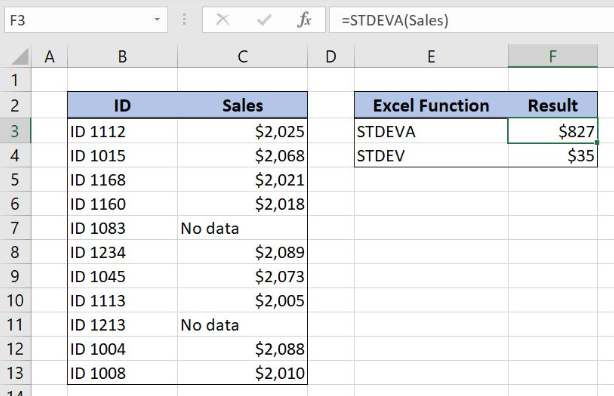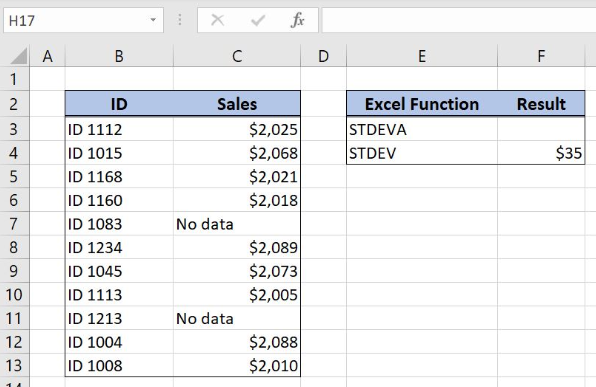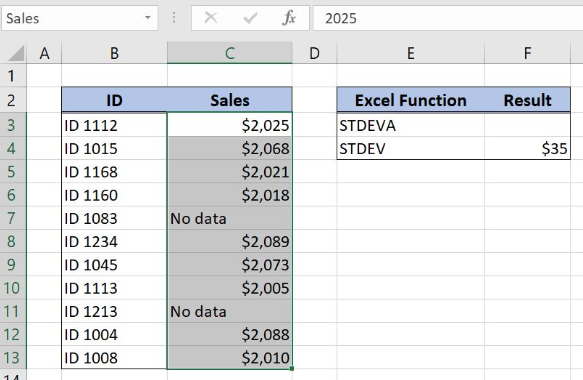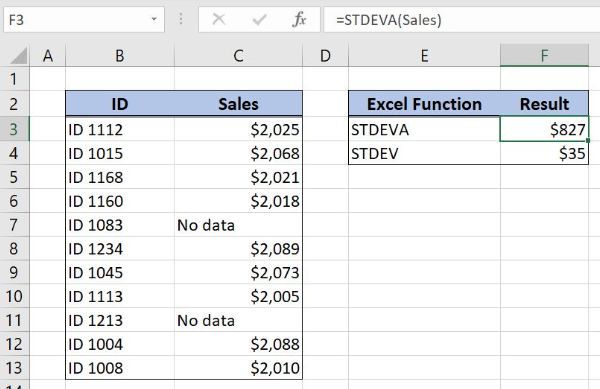Get instant live expert help with Excel or Google Sheets“My Excelchat expert helped me in less than 20 minutes, saving me what would have been 5 hours of work!”

#### Post your problem and you’ll get Expert help in seconds.

Your message must be at least 40 characters
Our professional Expert are available now. Your privacy is guaranteed.

# Excel STDEVA Function

Excel allows us to calculate the standard deviation of the sample with Excel STDEVA Function. Comparing to STDEV function, STDEVA function takes into account logical and text values in the calculation. The text is interpreted as 0, TRUE as 1 and FALSE as 0. This step by step tutorial will assist all levels of Excel users in getting the standard deviation of the sample including text and logical values.Figure 1. Calculate the standard deviation of a sample with STDEVA function

## Syntax of the STDEVA Function

`=STDEVP(value1, [value2], ...)`

The parameters of the STDEVP function are:

• value1, [value2] – the values in the data range for calculating the standard deviation for the sample

## Setting up Our Data for STDEVA Function

Our first table consists of 2 columns: “ID” (column B) and “Sales” (column C). The second one has the value of standard deviation calculated with STDEV function with the result placed in the cell F4. The idea is to get the standard deviation of sales data based on the sample with STDEVA function and to place the result in the cell F3.Figure 2. Table structure for STDEVA Function

## Get Standard Deviation Using STDEVA Function

We want to get the standard deviation of the data in the “Sales” column. In order to make the formula more clear, we will create a named range Sales for cell range B3:B13.

To create a named range we should follow the steps:

• Select the cell range that should be named
• Click on the name box in Excel
• Write the name for the cell range and press enterFigure 3. Creating a named range Sales for column “Sales”

The formula for getting the standard deviation for sales data is:

`=STDEVA(Sales)`

The value1 in the STDEVA function is the named range Sales.

To apply the STDEVA function we need to follow these steps:

• Select cell F3 and click on it
• Insert the formula: `=STDEVA(Sales)`
• Press enterFigure 4. Get the standard deviation of the sample including text and logical values

STDEVA function calculates the standard deviation of the sample. This function evaluates logical values and text in the calculation of the standard deviation. In our example text is interpreted as zero and the formula result in the cell F3 is \$827. This result means that sales values differ \$827 from the mean of the sales data range.

Most of the time, the problem you will need to solve will be more complex than a simple application of a formula or function. If you want to save hours of research and frustration, try our live Excelchat service! Our Excel Experts are available 24/7 to answer any Excel question you may have. We guarantee a connection within 30 seconds and a customized solution within 20 minutes.